# Python | Angle Spectrum using Matplotlib

Python | Angle Spectrum using Matplotlib: In this tutorial, we are going to learn how to plot angle spectrum using matplotlib?
Submitted by Anuj Singh, on July 22, 2020

The angle spectrum is one the most used functions in signal processing and Matplotlib has provided a function for plotting Angle Spectrum directly i.e. matplotlib.pyplot.angle_spectrum().

The following are the few examples to illustrate how to use the angle spectrum in matplotlib.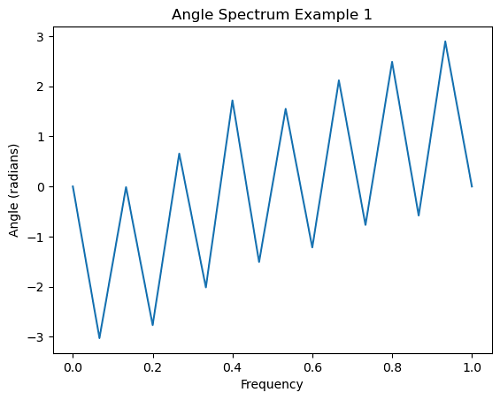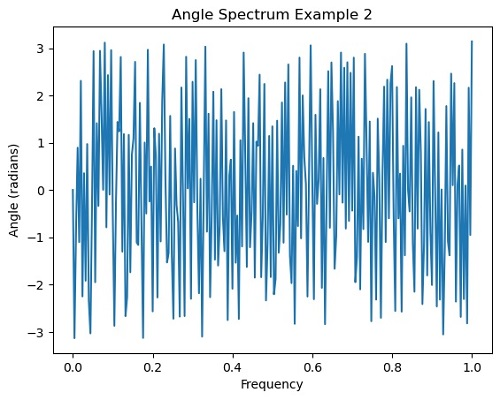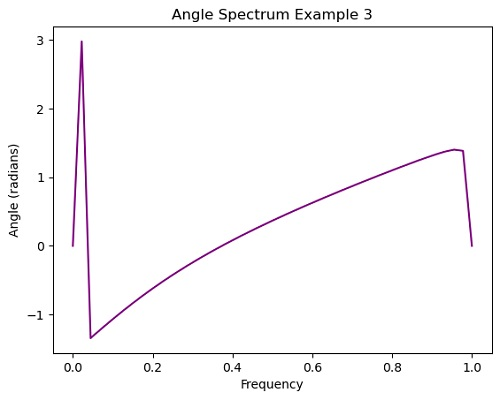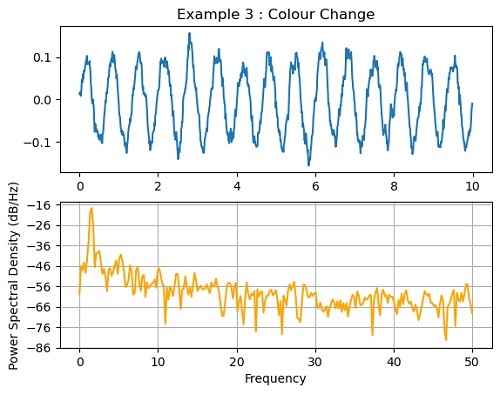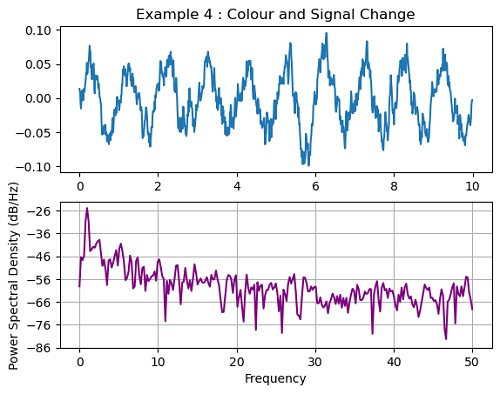## Python code for angle spectrum using matplotlib

```import numpy as np
import matplotlib.pyplot as plt

#Example 1
plt.figure()
pts = np.random.rand(30)*.2
pts[[3, 14]] += .8
plt.angle_spectrum(pts)
plt.title('Angle Spectrum Example 1')

#Example 2
plt.figure()
x = np.random.randint(40,80,500)
plt.angle_spectrum(x)
plt.title('Angle Spectrum Example 2')

#Example 3
plt.figure()
x = np.arange(60,150)
plt.angle_spectrum(x, color='purple')
plt.title('Angle Spectrum Example 3')
```

Output:

```Output is as figure
```

Languages: » C » C++ » C++ STL » Java » Data Structure » C#.Net » Android » Kotlin » SQL
Web Technologies: » PHP » Python » JavaScript » CSS » Ajax » Node.js » Web programming/HTML
Solved programs: » C » C++ » DS » Java » C#
Aptitude que. & ans.: » C » C++ » Java » DBMS
Interview que. & ans.: » C » Embedded C » Java » SEO » HR
CS Subjects: » CS Basics » O.S. » Networks » DBMS » Embedded Systems » Cloud Computing
» Machine learning » CS Organizations » Linux » DOS
More: » Articles » Puzzles » News/Updates